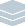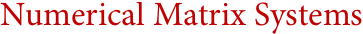Wolfram Archive

# Numerical Matrix Systems(Matlab, O-Matrix, Scilab, Octave, ...)
Evolving from the Fortran subroutine libraries of the 1970s, numerical matrix systems are based on the idea of representing all computations in terms of machine-precision numerical matrices. Mathematica takes a vastly more general approach, allowing it to integrate a fundamentally broader and deeper range of capabilities—yet still routinely outperform specialized numerical matrix systems even on basic matrix operations.
With its broader modern approach, Mathematica immediately allows a much higher level of computation—directly manipulating formulas and equations symbolically, validating and extending numerical precision, automatically selecting optimal algorithms, integrating active computation and interfaces into presentation-quality documents, and routinely handling data with arbitrarily general structure.
Particularly in recent years, Mathematica's unified design and unique integration of algorithms and methods from numerical, symbolic, geometric, discrete and other areas have allowed the creation of major new generations of algorithms which greatly extend the type and range of computations that can routinely be done—and make possible new levels of efficiency even in seemingly straightforward numerical tasks.
Long used in large-scale projects across many industries, Mathematica's scalable core language, support for modern web and grid deployment, dynamic interface creation capabilities and broad integration with external systems make it a uniquely productive development environment that provides a stable long-term platform for existing and future projects.
Numerical Matrix System Features in Mathematica:
Key Advantages of Mathematica Compared to Numerical Matrix Systems:
Interoperability with Numerical Matrix Systems:
Interesting Tidbits:
• Mathematica's state-of-the-art coverage of numerical matrix operations represents only 5% of its complete internal code base
• Wolfram Research has pioneered many numeric-symbolic hybrid algorithms
• A large fraction of Mathematica's numerical algorithms are based on original research at Wolfram Research